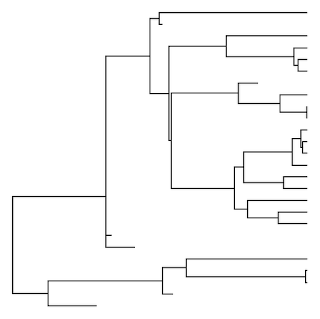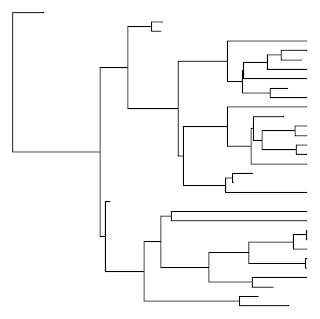## Tuesday, February 28, 2012

### Determining if the basal node of a tree leaves descendants from both its right & left daughters

A colleague contacted me last night to ask the following:

"Can you think of a quick way to test whether, in a simulated birth-death tree, both lineages descending from the basal node are represented in the extant taxa?"

In other words, can we distinguish:

> require(geiger)
> tr1<-birthdeath.tree(b=1,d=0.3,taxa.stop=20)
> plotTree(tr1,ftype="off",pts=F)from:

> tr2<-birthdeath.tree(b=1,d=0.3,taxa.stop=20)
> plotTree(tr2,ftype="off",pts=F)(aside from, say, by visual inspection)?

It immediately occurred to me that asking if both the right and left daughters of the root node have left descendants among the extant taxa is the same as asking if the MRCA of all the extant taxa in the tree was equal to the root node. By chance, in response to another user request I recently wrote a different function, findMRCA, that returns the MRCA for a list of species. All, then, that was needed was a function to pull out a list of the extant taxa in the tree. There is a couple of different ways I could envision doing this, but the following is what I settled on:

getExtant<-function(tree,tol=1e-8){
H<-nodeHeights(tree)
tl<-max(H)
x<-which(H[,2]>=(tl-tol))
y<-tree\$edge[x,2]
y<-y[y<=length(tree\$tip)]
z<-tree\$tip.label[y]
return(z)
}
getExtinct<-function(tree,tol=1e-8)
setdiff(tree\$tip.label,getExtant(tree,tol))

The way this function works is by first using nodeHeights to get the height above the root of all the internal and terminal nodes in the tree. It then gets the node numbers of the extant taxa by finding all the terminal nodes that are (within a certain tolerance) equal in height to the maximum height of the tree. These node numbers are then used to pull the tip labels of all the extant taxa from tree\$tip.label.

Having loaded the above function into memory, we can then ask if the node number of the root (which is always equal to length(tree\$tip)+1) is equal to the MRCA of the extant taxa in the tree as follows:

> require(phytools) # phytools 0.1-7 or above
> (length(tr1\$tip)+1)==findMRCA(tr1,getExtant(tr1)) # expect TRUE
 TRUE
> (length(tr2\$tip)+1)==findMRCA(tr2,getExtant(tr2)) # expect FALSE
 FALSE

Cool.